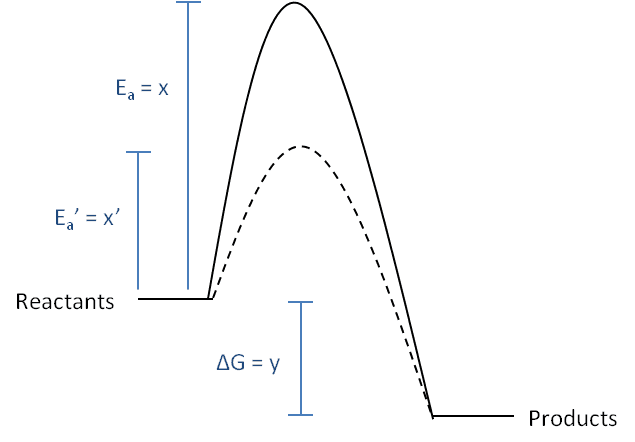# Catalyst effect on equilibria

We are taught that adding a catalyst to an equilibrium system does not alter the position, just the rate of equilibrium attainment, because it increases the forward and backward rate by the same amount. Why and how can that be? The activation energies will be different for the forward and backward direction. Does a change in forward/backward pre-exponential factors somehow exactly cancel the difference in Ea? Seems hard to believe. Or is it some outcome from the Boltzmann distribution curve? Or some other reason??!!

It doesn't affect equilibrium. However, a change in temperature, which is sometimes referred to as a catalyst, will change the equilibrium.

The equilibrium composition depends upon the temperature, pressure, and the Gibbs energies of each species. A chemical catalyst will not change any of these things.

If the activation energies are very large, the system will have difficulty reaching equilibrium, and it may not occur, but the equilibrium is defined as the lowest possible energy state for the system.

Ygggdrasil
Gold Member
Consider the following reaction with free energy change, y:The uncatalyzed reaction has activation energy x while the catalyzed reaction (shown in dotted lines) has an activation energy x'.

a) What is the activation energy for the reverse reaction (products --> reactants) in the absence of a catalyst? What is the activation energy for the reverse reaction in the presence of a catalyst?

b) The pre-exponential factors for the forward and reverse reactions are A and B, respectively. Write an expression for the forward and reverse rates in terms of A, B, x, and y.

c) What is the ratio of the forward rate / reverse rate for the uncatalyzed reaction? What is the ratio of the forward rate / reverse rate for the catalyzed reaction? How do they compare?

That's brilliant! Yes I had not taken the time to work it through to realise that the rate constant ratios are power functions which will cancel down. If we take say x = 5, x' = 2, y =4, then just using these data, 5/9 uncat does not equal 2/6 cat ... but e^5/9 = e^2/6 = e^-4!!

So we obtain k forward / k backward = A/B e ^y/RT. At equilibrium, kfwd / kbwd = K, ΔG = 0, and y = ΔGθ giving us K = A/B e^ΔGθ/RT ... which if we take natural logs and rearrange becomes ΔGθ = RT InK InB/A ... which is almost the same as the standard equation ΔGθ = -RT InK!! We have lost a minus sign and gained a constant - any final thoughts as to how to reconcile?

PS I will be posting a question on haloalkane rates shortly (how's your kinetics?!!) and wanted to draw a graphic similar to yours - how did you produce that for this thread - which software or method?

Thank you very much indeed!

Only temperature affects equilibrium I think...

Only temperature affects equilibrium I think...

Pressure does too if you're dealing with gas phase reactions and different molar amounts of reactants and products. Also if you had different specific volumes in a liquid/solid phase reaction between the reactant and product side, you'd see a changing pressure affect the equilibrium.

... obviously I meant e^5/e^9 = e^2/e^6 = e^-4!!

... if you get a minute dear ygggdrasil to let me know your graphic secret (!) I would be much obliged!

Ygggdrasil
Gold Member
... if you get a minute dear ygggdrasil to let me know your graphic secret (!) I would be much obliged!

I made the graphic in powerpoint and exported the image as a .png file. Powerpoint is surprisingly useful for making simple figures.

I"ll answer the rest of your question later as I have to look up a few things first.

Ygggdrasil
Gold Member
So we obtain k forward / k backward = A/B e ^y/RT. At equilibrium, kfwd / kbwd = K, ΔG = 0, and y = ΔGθ giving us K = A/B e^ΔGθ/RT ... which if we take natural logs and rearrange becomes ΔGθ = RT InK InB/A ... which is almost the same as the standard equation ΔGθ = -RT InK!! We have lost a minus sign and gained a constant - any final thoughts as to how to reconcile?

Here instead of using the Arrhenius equation (which is just an empirical relationship), it's better to use the results derived from transition state theory. According to transition state theory, the rate constants k for reactions are given by the Eyring equation:
$$k = \frac{k_BT}{h}e^{-\Delta G^*/RT}$$ where kB is the Boltzman constant, h is Planck's constant, R is the gas constant, and ΔG* is the free energy of activation.

For the example given in my post, we get the following forward and reverse rate constants:
$$k_f = \frac{k_BT}{h}e^{-x/RT}$$$$k_r = \frac{k_BT}{h}e^{-(x+y)/RT}$$ The ratio of the forward and reverse rate constants is then:
$$\frac{k_f}{k_r} = e^{y/RT}$$Substituting the equilibrium constant K = Kf/kr, we get: y = RT ln(K)

Here, the reaction is clearly exergonic (ΔG < 0), so y = -ΔGo, and with this substitution, we recover the relationship between the free energy change of the reaction and the equilibrium constant:

ΔGo = -RT ln(K)

Last edited:
Thats really helpful thanks so much!
I have posted a question on haloalkane rates of reacton - hope you can take a look!
Thanks again